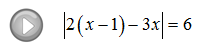## Algebra

### Absolute Value Equations

Absolute value, by definition, is the distance from zero on a number line.

If we have |x| = 3 then the question is, “what number can x be so that the distance to zero is 3?” There are two solutions to this question -3 and 3.In general,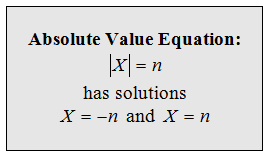Here n is a positive integer and X represents an algebraic expression called the argument of the absolute value. To solve these equations, set the argument equal to plus or minus n and solve the resulting equations.

Solve.This technique requires us to first isolate the absolute value. Apply the usual steps for solving to obtain the absolute value alone on one side of the equation, and then set the argument to plus or minus n.Solve.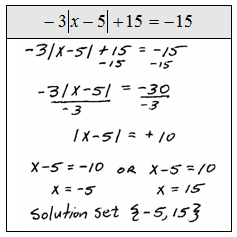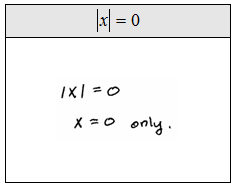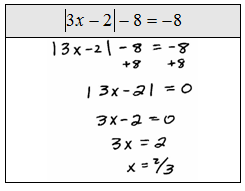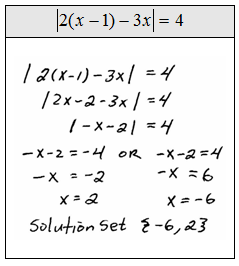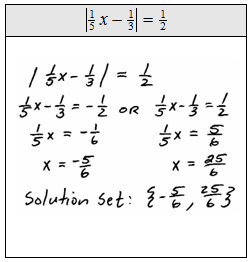When two absolute values are equal we can set the argument of one equal to the argument of the other.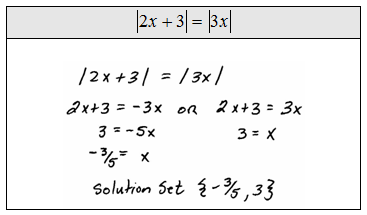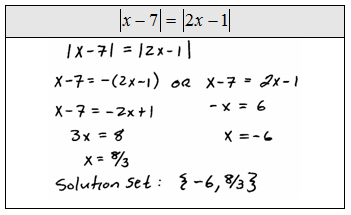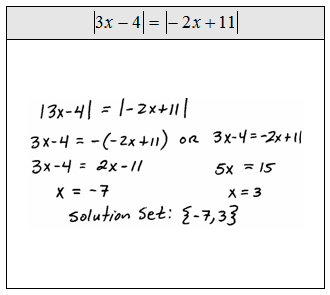Tip: When in doubt of a solution, check to see if it solves the original equation.  For example, check that {-7, 3} is the solution set for,Notice that both numbers solve the original equation and therefore we have verified they are in the solution set.